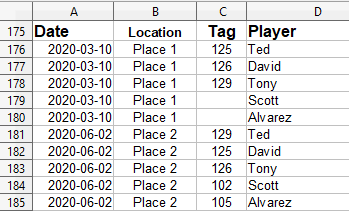# Why does Array Function get reset to non-Array Function?

I have an .ods file of ~1MB, ~50 sheets. Recently with LO6.2 I noticed that two different sheets containing array functions that reference a same third sheet were showing incorrect values.

I upgraded to LO6.3 and the same problem was present.

I figured out that the curly braces were missing from the formulas in both sheets, i.e. the array formulas had reverted to standard formulas. There were other array formulas in the doc that where still correct but they didn’t reference the same sheet as the ones that had broken.^^ “Scores” sheet. (Contains date sorted list of Players and Tags; where Tags my be reassigned on subsequent dates)

Formula in Sheet1 to get most recent “Tag” number given Player in B2

``````{=INDEX(Scores.\$C\$1:\$C\$437, MAX(ROW(Scores.\$D\$1:\$D\$437)*(Scores.\$D\$1:\$D\$437=B2)*(Scores.\$C\$1:\$C\$437>0)))}
``````

Formula in Sheet2 to get most recent “Player” given Tag number in M2

``````{=INDEX(Scores.\$D\$1:\$D\$437, MAX(ROW(Scores.\$C\$1:\$C\$437)*(Scores.\$C\$1:\$C\$437=M2)*(Scores.\$C\$1:\$C\$437>0)))}
``````

I had been editing the Scores sheet to move a bunch of rows around by inserting blank rows, then cutting/pasting rows to move them. All this was within the range of rows 1:437. I’m 99% sure that’s what broke the array formulas and reverted them back to standard formulas.

I say “99%” because I fixed the array formulas, did some more moving rows editing in Scores and the Sheet1 formulas broke again. Interestingly, this time the Sheet2 formulas did not break.

The problem is that I can’t seem to make a reproducible test case for this.

I’ve been using this doc in OpenOffice and LibreOffice for going on six years now and never noticed this problem until this week.

First question: am I doing something wrong?

Second question: any suggestions on how to get a reproducible test case to submit a bug?

Thanks.
ArrayFormBug.ods

If you made changes to the array formulas - did you finalize the edit using `CTRL+SHIFT+ENTER` instead of `ENTER`? If yes - then that’s the cause of your problem (LibreOffice Help - Array Functions)

That’s not the issue. The array formulas were originally input with CTRL+SHIFT+ENTER. The cell formulas showed with {}'s and the cell values were correct.

I then edited (moved rows around) a different sheet with no array formulas, but the edited rows were in the range of the array formulas of a different sheet. These edits caused the array formulas to convert to normal/non-array formulas without me touching the array formulas.

I then fixed the array formulas by re-entering with CTRL+SHIFT+ENTER and all was right in the world… until I did some more row moving and some of the array formulas broke again the same way as before.

Much of array formulas can be involved in the SUMPRODUCT() function, what avoids the need to enter with [Ctrl+Shift+Enter]if they has a number as result.

SUMPRODUCT can’t be used in this situation as I do not want to add the elements of the array, but instead find the MAX value of them. If there were a MAXPRODUCT function I’d use that.

The data is an unsorted column of either names or numbers with potentially many duplicates. I’m looking for the last occurrence in the column of a specific value.

E.g. if I pass in Player “Scott” I want to return the Tag 102 (on row 184), not a blank (on row 179). In the other usage if I want look up who has Tag 126 it should return “Tony” (on row 183), not “David” (on row 177).

SUMPRODUCT can’t be used in this situation as I do not want to add

Please note: Function `SUMPRODUCT()` often is used just to force array evaluation and to avoid array formulas using `CTRL+SHIFT+ENTER`. Example:

`{=COUNT(IF(A1:A10<>0))}`
`=SUMPRODUCT(COUNT(IF(A1:A10<>0)))`

both count the number of cells having a non-zero value in the range `A1:A10`

SUMPRODUCT with MAX can be used, if you attach a sample file someone can take a look on how to do it.

I’ve uploaded a .ods stripped down to the relevant sheets and data.

=== The “Scores” sheet contains the list of players and tags across multiple events/dates. On a given date a player earns a physical numbered tag. These tags are exchanged from week to week based on event scores.

=== The “Tags” sheet is a summary of Tags by number to quickly see who has which numbered tag.

=== The “Players” sheet is an alphabetical list of Players to quickly see which Tag a given player has.

It’s all great if someone can come up with a more efficient set of formulas. However… I started this thread because of what I believe is a BUG where the array “property” of formulas is lost when editing the data at which the array formulas point.

I have been dealing with the same issue for years; my workbook is 150 KB with 7 tabs, one of which has just over 120 array formulas referencing data in another tab. The “Raw Data” tab that the array formulas reference is frequently modified with row inserts and the occasional Paste Values to transfer values from one of the utility tabs. Often when I finish working in the file, I’ll check the tab with the array formulas before closing. Many times the formulas are intact, but sometimes the array flag has mysteriously reset. (And when it does, all 120 array formulas are affected at the same time.)

I can confirm that wrapping the formula in SUMPRODUCT obviates the need for the array formula mode. Nevertheless, it is a bug in LibreOffice. This is the only content I could find on a Google search that calls out the bug. Unfortunately, I too am unable to construct a reproducible testcase file, because I cannot tell what triggers the bug.